A100 Dan Swearingen
Homework 2, Due Tuesday, September 24

Chapter 1

Pages 53-54, Questions For Review:

1. What is the celestial sphere?

The celestial sphere was originally part of the ancient model of the universe with the Earth at the center and the stars riding on a crystal sphere (the celestial sphere) which enclosed the Earth. Today, the concept of the celestial sphere is how we map the sky by projecting the Earth's poles and equator onto the sky to establish coordinates and projecting the angular position of objects in the sky using these coordinates.

2. What is a constellation?

A constellation is a recognized grouping of stars in the sky. Astronomers use constellations to divide the sky into 88 regions.

3. What is the Zodiac?

The Zodiac is the band around the sky where the Sun, Moon, and planets may be found. In the Zodiac there are twelve constelleations dividing the Zodiac into twelve regions.

4. What is the Ecliptic?

The Ecliptic is the appearent path of the Sun around the sky caused by the Earth's annual motion around the Sun.

5. Where on the Celestial Sphere would you look for the planets?

By its definition, the Zodiac is where in the sky you should look for the planets.

6. How long does it take for the moon to go through a cycle of phases?

The moon takes 28 days to go around the Earth. However, it takes about 30 days to go from full moon to the next full moon.

9. If you see a bright "star" in the sky, how could you tell whether it is a star and not, for example, Venus?

To be sure it is not a planet you must look at it over several weeks or months and see if it moves against the background stars. If it does not move it is a star. If it moves it is a planet (or asteroid!).

Page 54, Thought Questions and Problems:

1. If you were standing on Earth's equator, where would you look to see the north celestial pole?

The north celestial pole (NCP) is the north pole of the sky. Like the earth's north pole, the NCP is 90 degrees from the equator. If you are standing at the earth's equator then (by definition) the celestial equator (CE) will run east to west passing through straight overhead. The NCP will be 90 degrees from the CE, so the NCP will be 90 degrees from straight overhead -- on the North horizon.

Could you see this pole from Australia?

No. When you are south of the equator the 180 degrees of sky which you can see do not include the direction to the NCP. See the figure below: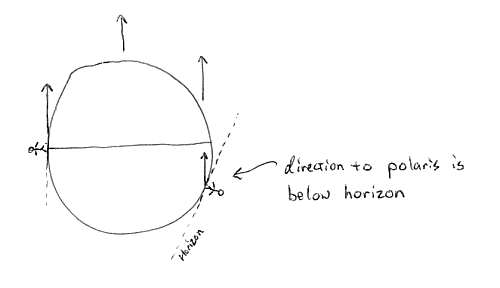4. Can you think of an astronomical reason why the zodiac may have been divided into 12 signs rather than 8 or 16?

The moon takes about 30 days to go from full to full again. So there are about 12 full moons each year. With 12 signs in the zodiac the sun will advance one sign each successive full moon.

7. Why does the position of sunrise along the eastern horizon change during the year?

The position of sunrise changes over the course of the year because the earth's axis is tilted 23.5 degrees and that tilt points the same direction as the earth moves around the sun as shown below: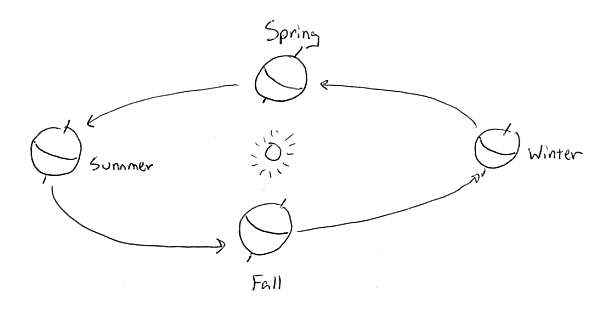This tilt and the change in position of the earth as it goes around the sun causes the sun's position at sunrise to change with the season. When it is the first day of summer in the northern hemisphere, the north pole of the earth is tilted towards the sun: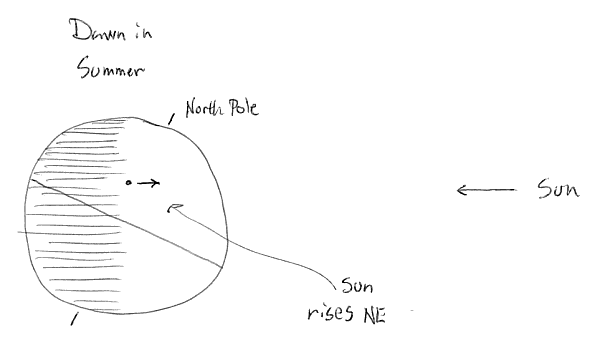When it is the first day of Fall in the northern hemisphere the north pole is tilted neither towards or away from the sun (in the drwaing below the north pole is tilted directly towards the viewer):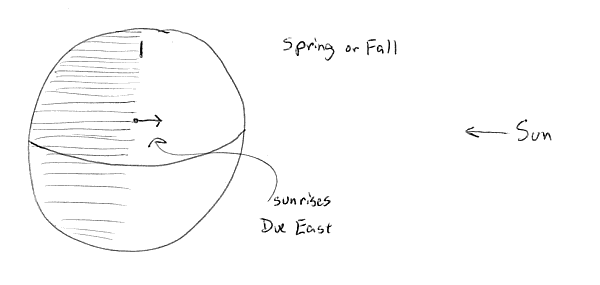When it is the first day of winter in the northern hemisphere the earth's north pole is tiltted away from the sun: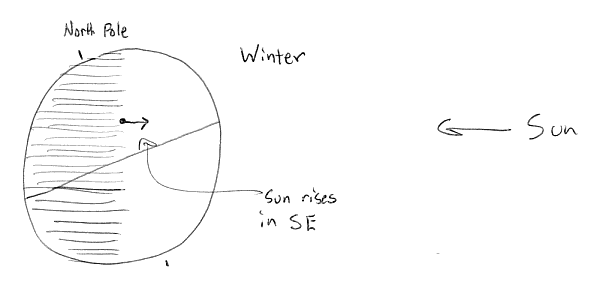So the position of sunrise moves from the southeast, to due east, to northeast and back again as the earth moves around the sun.

9. Suppose a planet were found with an orbital period of 64 years. How might you estimate its distance from the sun?

You can estimate the semi-major axis, or average distance from the sun, of this planet's orbit by using Kepler's Third Law where the period, P is 64 years: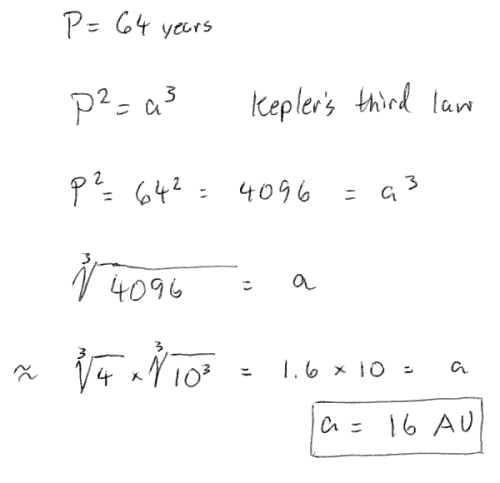If the planet's orbit is circular, what is its radius?

By Kepler's First Law, all orbits are ellipses. Here is an example of a very elliptical orbit: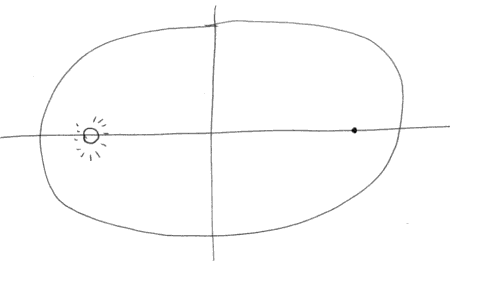However, a circle is just the special case of an ellipse with zero eccentricity: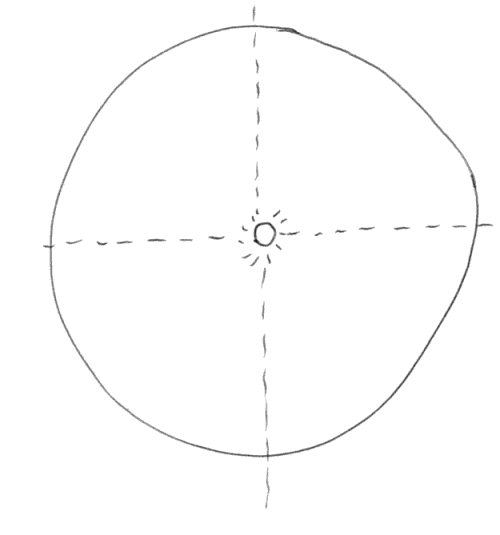It can be seen that the semi-major axis for a circular orbit will be the same a the radius of the circle, so the answer is that if the orbit is circular, its radius will be 16 AU.

Essay 1

Page E1-10, Questions:

1. Approximately where would you look for Mercury in the sky at 7 p.m.?

From our point of view, Mercury is never more than 28 degrees away from the sun in our sky. As the sun nears setting around 7 p.m. I would look back (westward) along the sun's path to see Mercury: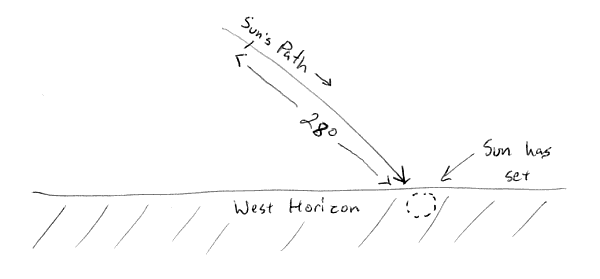3. If a planet is at opposition and you see it straight overhead, what time of night must it be?

Opposition is when a planet is opposite the sun, or 180 degrees away from the sun from our point of view. So a planet in opposition will be high overhead when we are turned 180 degrees away from the sun which is midnight.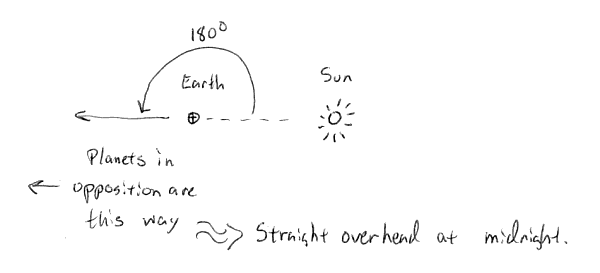Chapter 2

Page 74

Questions For Review:

4. How does mass differ from weight?

Mass is a property of matter which makes it necessary to apply a force in order to accelerate matter. Weight is a force caused by the acceleration a mass is experiencing. Your bathroom scale measures how much force your body is pressing down on the scale with.

5. If your mass is 70 kg on earth, what is it on the moon?

Your mass will be 70 kg, assuming you did not gain or lose mass during your trip to the moon. Your weight will be about one-sixth what it was on earth.

Thought Questions and Problems:

4. How much bigger is the earth's gravitational force on the moon than the moon's gravitational force on the earth? Think about Newton's third law of motion before answering this.

There are two ways to see this: To calculate the force of gravity on the Earth from the moon you would set up Netwton's law of gravity with the masses of the earth and the moon and the distance between them: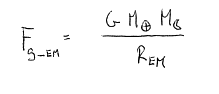Similarly, if you wanted to calculate the force of gravity on the moon from the earth you would set up Netwton's law of gravity with the masses of the earth and the moon and the distance between them:But these are the same! Further, Newton's third law says that for any force there is an equal and opposite reaction. This also tells us that the force the earth exherts on the moon is the same as the force the moon exherts on the earth.